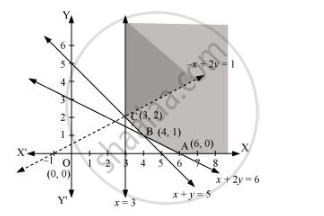Share

# Show that the Minimum of Z Occurs at More than Two Points Maximise Z = – X + 2y, Subject to the Constraints X ≥ 3, X + Y ≥ 5, X + 2y ≥ 6, Y ≥ 0. - CBSE (Science) Class 12 - Mathematics

ConceptLinear Programming Problem and Its Mathematical Formulation

#### Question

Show that the minimum of Z occurs at more than two points

Maximise Z = – x + 2y, subject to the constraints x ≥ 3, x + y ≥ 5, x + 2y ≥ 6, y ≥ 0.

#### Solution

The feasible region determined by the constraints, x ≥ 3, x + y ≥ 5, x + 2y ≥ 6, y ≥ 0. is as follows.It can be seen that the feasible region is unbounded.

The values of Z at corner points A (6, 0), B (4, 1), and C (3, 2) are as follows

 Corner point Z = −x + 2y A(6, 0) Z = − 6 B(4, 1) Z = − 2 C(3, 2) Z = 1

As the feasible region is unbounded, therefore, Z = 1 may or may not be the maximum value.

For this, we graph the inequality, −x + 2y > 1, and check whether the resulting half plane has points in common with the feasible region or not.

The resulting feasible region has points in common with the feasible region.

Therefore, Z = 1 is not the maximum value. Z has no maximum value

Is there an error in this question or solution?

#### APPEARS IN

Solution Show that the Minimum of Z Occurs at More than Two Points Maximise Z = – X + 2y, Subject to the Constraints X ≥ 3, X + Y ≥ 5, X + 2y ≥ 6, Y ≥ 0. Concept: Linear Programming Problem and Its Mathematical Formulation.
S